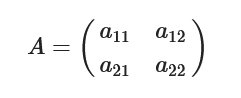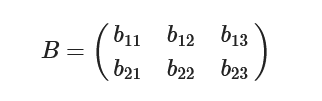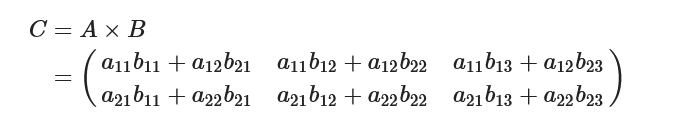×

Search anything:

# Matrix Multiplication in C

#### C Programming AlgorithmsIn this article, we are going to perform the matrix multiplication operation using C language. We will also be learning how to optimize it using Strassen's algorithm.

Table of Content:

• Introduction
• Concept of Matrix Multiplication Operation
• Matrix Multiplication in C
• Time Complexity
• Cache Performance
• Optimizing Matrix Multiplication using Stassen's Method
• Conclusion

# Introduction

A matrix is a rectangular array of numbers, symbols, or expressions, arranged in rows and columns.
For example, the following is a matrix with 2 rows and 3 columns:

[ 1 3 5 ]
[ 2 4 6 ]

Each element in the matrix is referred to by its row and column indices. For example, the element in the first row and second column is 3. Matrices are often used in mathematics, science, and engineering to represent systems of equations, data, and other types of information. They can be manipulated and analyzed using a variety of mathematical operations.

# Concept of Matrix Multiplication Operation

Suppose A is an m×n matrix, B is an p×q matrix, then the m×q matrix C is called the product of matrix A and matrix B, denoted as C=AxB

Let's consider the following product AB of matrix A with 2 rows and 2 columns B with 2 rows and 3 columns.

Input matrix 'A'and the matrix 'B'Product (multiplication) of# Matrix Multiplication in C

Idea :

Define the matrices A, B, and C as 2D arrays. Initialize the matrices A and B with some values, and initialize C to an array of all 0s.

Use three nested for loops to iterate over the elements of the matrices. The outer two loops will iterate over the rows and columns of the result matrix C, and the inner loop will iterate over the columns of A and rows of B.

Inside the innermost loop, use the loop indices to access the elements of the matrices A and B, and perform the matrix multiplication. Specifically, multiply the element of A at the current row and column of the inner loop by the element of B at the current column of the inner loop and row of the outer loop, and add the result to the element of C at the current row and column of the outer loops.

After the three loops have completed, the result matrix C will contain the product of the matrices A and B.

Optionally, print the result matrix C using another nested loop.

Implemented Code:

``````#include<stdio.h>

int main()
{
int a = {2,3,4,5,3,5};
int b = { 1,2,3,4,5,6 };
int c;
for (int i = 0; i < 3; i++)
{
for (int j = 0; j < 3; j++)
{
c[i][j] = 0;
for (int k = 0; k < 2; k++)
c[i][j] += a[i][k] * b[k][j];
}
}

printf("The result of multiplying matrix 1 and matrix 2 is:\n");
for (int i = 0; i < 3; i++)
{
for (int j = 0; j < 3; j++)
{
printf("%d\t", c[i][j]);
}
printf("\n");
}
return 0;
}
``````

Output :

``````The result of multiplying matrix 1 and matrix 2 is:
14	19	24
24	33	42
23	31	39
``````

Code Explanation :

This code will compute the product of two matrices A and B, and store the result in a new matrix C.
The first loop iterates in rows for matrix A, second loop iterates aroucolumns in matrix B.
The third loop works to put the multiplied values, add them and the result goes inside matrix C.

The matrices A and B must have compatible dimensions for the multiplication to be possible (in this case, the number of columns of A must be equal to the number of rows of B). The resulting matrix C will have the same number of rows as A and the same number of columns as B.

Note that matrix multiplication is not commutative, meaning that the order in which the matrices are multiplied matters. For example, A * B is not necessarily equal to BxA.

# Time Complexity

TC - O(n^3)
Reason - 3 for loops nested

# Cache Performance

The cache performance of the matrix multiplication code in C depends on the access patterns of the matrices A, B, and C, and the size and organization of the cache memory. In general, the performance can be improved by optimizing the access patterns to take advantage of the cache.

One way to improve the cache performance is to use a row-major layout for the matrices, which means that the elements in each row are stored in contiguous memory locations. This allows the elements in a row to be accessed with fewer cache misses, since they are likely to be stored in the same cache line.

To further optimize the performance, it is important to access the elements of the matrices in a contiguous order. For example, instead of accessing the elements of C in the order C, C, C, ..., it may be more efficient to access the elements in the order C, C, C, ... This will result in fewer cache misses, since the elements in each column of C will be accessed contiguously.

Another optimization is to use blocking or tiling techniques, which break the matrices into smaller blocks and perform the multiplication on the blocks separately. This can reduce the number of cache misses by allowing the blocks to fit in the cache more efficiently.

# Optimizing Matrix Multiplication using Stassen's Method

The Strassen algorithm is a fast matrix multiplication algorithm that was developed by Volker Strassen in 1969.

It is an improvement over the standard matrix multiplication algorithm, which has a time complexity of O(n^3) for multiplying two n x n matrices. The Strassen algorithm reduces the time complexity to O(n^2.807), which is faster for large matrices.

The basic idea behind the Strassen algorithm is to divide each matrix into four smaller submatrices, and recursively multiply the submatrices using a series of simpler matrix operations. These operations are based on the following identities:

A x B = (A + D) x (B + C) - C x D - A x B
A x (B + C) = A x B + A * C

where A, B, C, and D are submatrices of the original matrices.

Idea Explained:

The algorithm works by dividing the matrices into submatrices of size n/2 x n/2, and recursively multiplying the submatrices until the base case of n = 1 is reached. At this point, the submatrices can be multiplied using the standard matrix multiplication algorithm. The result is then combined using the identities above to obtain the final result.

Implemented Code:

``````#include<stdio.h>

int main(){

int a, b, c, i, j;

int m1, m2, m3, m4 , m5, m6, m7;

printf("Enter the 4 elements of first matrix: ");
for(i = 0;i < 2; i++)
for(j = 0;j < 2; j++)
scanf("%d", &a[i][j]);

printf("Enter the 4 elements of second matrix: ");
for(i = 0; i < 2; i++)
for(j = 0;j < 2; j++)
scanf("%d", &b[i][j]);

printf("\nThe first matrix is\n");
for(i = 0; i < 2; i++){
printf("\n");
for(j = 0; j < 2; j++)
printf("%d\t", a[i][j]);
}

printf("\nThe second matrix is\n");
for(i = 0;i < 2; i++){
printf("\n");
for(j = 0;j < 2; j++)
printf("%d\t", b[i][j]);
}

m1= (a + a) * (b + b);
m2= (a + a) * b;
m3= a * (b - b);
m4= a * (b - b);
m5= (a + a) * b;
m6= (a - a) * (b+b);
m7= (a - a) * (b+b);

c = m1 + m4- m5 + m7;
c = m3 + m5;
c = m2 + m4;
c = m1 - m2 + m3 + m6;

printf("\nMultiplication using Strassen's algorithm \n");
for(i = 0; i < 2 ; i++){
printf("\n");
for(j = 0;j < 2; j++)
printf("%d\t", c[i][j]);
}

return 0;
}
``````

Output:

``````Enter the 4 elements of first matrix: 1 2
3 4
Enter the 4 elements of second matrix: 6 7
8 9
The first matrix is

1	2
3	4
The second matrix is

6	7
8	9
Multiplication using Strassen's algorithm

22	25
50	57
``````

Time Complexity : O(n^2.807)